# Troops

The route is long 147 km and the first-day first regiment went at an average speed of 12 km/h and journey back 21 km/h. The second day went second regiment the same route at an average speed of 22 km/h there and back.

Which regiment will take route longer?

Result

#### Solution: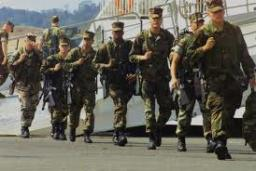We would be pleased if you find an error in the word problem, spelling mistakes, or inaccuracies and send it to us. Thank you!

Showing 1 comment:Math student
3qne=b=3wn8e b=Tips to related online calculators
Need help calculate sum, simplify or multiply fractions? Try our fraction calculator.
Check out our ratio calculator.
Do you have a linear equation or system of equations and looking for its solution? Or do you have quadratic equation?
Do you want to convert length units?
Do you want to convert velocity (speed) units?
Do you want to convert time units like minutes to seconds?

## Next similar math problems:

• 1.5 divided1.5 divided by 1 = w divided by 4
• Orchard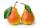Three-fifths of the orchard are apple trees, one-third of the trees are cherries. The remaining 5 trees are pears. How many trees are in the orchard?
• Unknown numberIdentify unknown number which 1/5 is 40 greater than one tenth of that number.
• Unknown numberI think the number - its sixth is 3 smaller than its third.
• The machineThe machine was in operation for 9 tenths (in a fraction) of eight hours of working time. How long has he been running?
• Determine the number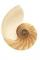Determine the number x that ?.
• Mushrooms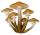For five days, we have collected 410 mushrooms. Interestingly every day we have collected 10 mushrooms more than the preceding day. How many mushrooms we have collected during 4th day?
• Father and sonFather is 48 years old, son 23. When will be the father twice older than a son?
• Two ages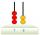Gabrielle is 9 years younger than Mikhail. The sum of their ages is 87. What is Mikhail's age?
• Eq-fracSolve the following equation with fractions: h + 1/3 =5/3
• Fraction + eqSolve following simple equation with fractions: -5/6(8+5b) = 75 + 5/3b
• Age ratioJanko is 14 years old. The age ratio of Janka and Zuzka is 2: 3. What was the ratio seven years ago?
• AgesFather is 36 years old, his daughter is 4 years old. Write down the ratio of the age of father and daughter. In what ratio will the ages of father and daughter after 4 years?
• MidnightHow many hours are, if the time that elapsed since 8:00 is 2/5 of the time that will past till midnight?
• Honza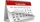Honza is 13 years old and Peter 21 years old. After how many years are their ages will be at ratio 7: 9?
• SeamstressThe seamstress cut the fabric into 3 parts. The first part was the eighth fabric, the second part was three-fifths of the fabric and the third part had a length of 66 cm. Calculate the original length of the fabric.
• Tourist routeHow long is the tourist route when tourists crossed four-sevenths of the way on foot, crossed the bus twice less than on foot and passed the last 14 kilometers by boat.